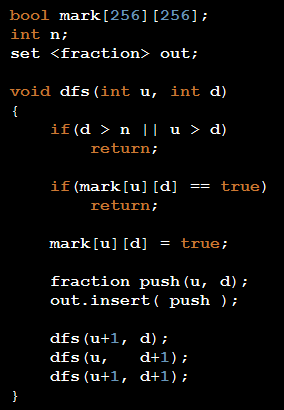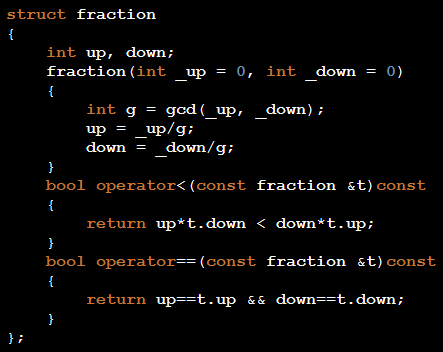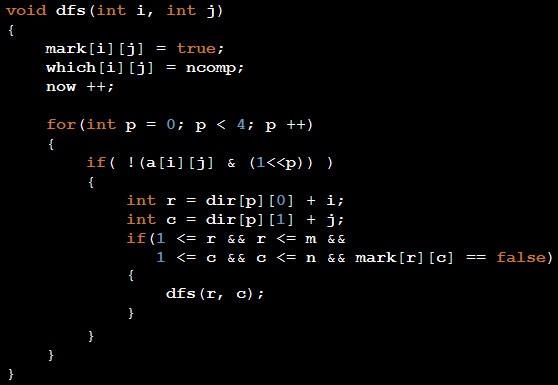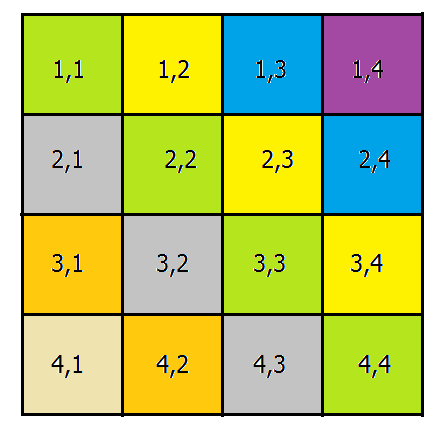## Thursday, January 10, 2013

### USACO - Ordered Fractions

One way to solve this problem is to construct all possible fractions, reduce all of them as possible, sort them and print them.

Another way is to use DFS.Lets start with (0,1) and see what can we build? (Check it yourself)
and fraction is something like this :### USACO - The Castle

Think about problem, what does the problem wants? We have a grid and we want to find the number of connected components in this grid, the largest component before breaking a wall, and the largest component after breaking a wall, we also need the wall itself.

To find connected components one can easily use DFS.
int dir = {{0, -1}, {-1, 0}, {0, 1}, {1, 0}};
To deal with neighbors I have this array 'dir', a[i][j] is the value of cell (i, j), I want to know whether I can go to the other cells connected to (i, j) or not.After DFS, I iterate over cells as the problem statement wants and check it's neighbors, if its neighbor is in another component I check the size of the merged component to the maximum size found.

### USACO - Checker Challenge

If you are familiar with n-queen problem, when you are reading the problem statement you will remember that problem, Yes we are facing a n-queen problem, we want to count different ways to put n queens in a n*n board.

For those not familiar with n-queen problem

The eight queens puzzle is the problem of placing eight chess queens on an 8×8 chessboard so that no two queens attack each other. Thus, a solution requires that no two queens share the same row, column, or diagonal. The eight queens puzzle is an example of the more general n-queens problem of placing n queens on an n×n chessboard, where solutions exist for all natural numbers n with the exception of 2 and 3.

We have to construct solutions, but how? We know that in each row and each column we have to put just one queen, the same thing applies to the diagonals.
So we start building solutions from row 1, we place one queen in row 1 column  j, for each j, recursively we put queens in appropriate columns. When we are putting a queen in column j, how do we know that this is an appropriate column? I have an array named bool column[n], which states whether there is a queen in column j (which has been put there in the previous rows.) This array suffices to check column condition, Row condition is already satisfied, because we are iterating over rows, but what about diagonals?But how to mark diagonals so that we can check it in O(1),
Take a look at these diagonals, what is the relation between (row,column) in each diagonal. I write diagonals here :
4,1 -> column-row is -3
3,1 | 4,2 -> column-row is -2
2,1 | 3,2 | 4,3 -> column-row is -1
1,1 | 2,2 | 3,3 | 4,4 -> column-row is 0
1,2 | 2,3 | 3,4 -> column-row is 1
1,3 | 2,4 -> column-row is 2
1,4 -> column-row is 3

What about other kind of diagonals, I mean diagonals like /?
1,1 -> column+row is 2
2,1 | 1,2 -> column+row is 3
3,1 | 2,2 | 1,3 -> column+row is 4
4,1 | 3,2 | 2,3 | 1,4 -> column+row is 5
4,2 | 3,3 | 2,4 -> column+row is 6
4,3 | 3,4 -> column+row is 7
4,4 -> column+row is 8

So I have two other arrays diag1, diag2 which is used to mark each diagonal.